Designing MOSFET Switch Technically Comprehensive

MOSFET is not well discussed in the undergrads; much more how to designing MOSFET switch. MOSFET stands for metal oxide semiconductor field effect transistor. Actually it is easy to use than BJT if we are talking as a switch circuit. Nowadays MOSFET is very popular in circuit designs such in automotive, power supply and general electronics as a low side and high side driver. It is a voltage controlled device so it is easy to bias to become a switch. There are two options in designing MOSFET switch. One is low side driving and other is high side driving. In the former, when the MOSFET saturates it will provide path to the circuit ground. For the latter, when the MOSFET saturates, it will provide path to the circuit supply.

In this article I will use a relay as a load because it has an inductive property that can possess challenge to the design. With this, the circuit is also called as relay driver.

By definition,a relay driver is a circuit that design to energize a relay coil to change the contact state. If we recall, a relay commonly has two contact states; open and close. Usually relays have normally open contact. This means that when the coil is not energized, the contact will stay open. The coil is can be driven by a BJT or a MOSFET but in this topic we will discuss the latter; MOSFET relay driver.

Some design engineers preferred the driving circuit to position in the lower side of the relay coil and this is called as low side driving. With the term itself, the driving circuit will be the one to provide the ground path of the relay coil for the current path to be closed.

The driving approach is not only limited to low side driving. High side driving is also possible. We will discuss the advantages and disadvantages of both low side and high side driving.

1. Designing MOSFET Switch Circuit Configuration

1.1. Low Side Driving

As mentioned above, there are two ways in designing MOSFET switch; either low side or high side. A common circuit for low side driving configuration is shown in Figure 1. The driving device S1 is located in the lower side of the relay coil such that it will provide a ground path when it is bias by V2. The device enclosed in green is the relay and the left side with a 10µH inductance is the coil while the right side is the contact.

S1 should be operated in the saturation region so that the voltage across the relay coil is high enough to satisfy the pickup or pull-in voltage requirement of the relay. By the way, a pickup voltage is a relay voltage requirement across its coil during startup to start operation. Usually, for a 12V rated relay the pickup voltage is 9V at nominal operating temperature. The pickup voltage will vary with operating temperature so better consider it in the actual design. In some applications such in power supply design, a small value of limiting resistor is inserted in series with the relay coil to at least minimize the coil current for efficiency reason but to keep the pickup voltage high enough to guarantee relay startup. In some application on the other hand such as automotive, efficiency is not a requirement so this limiting resistor is not a part of the design. Now, I have a question for you, if there is no limiting resistor in series with the relay coil, will the relay not damage since it is connected across the supply voltage? The answer is not. Relay coil has already a built-in internal resistance. This coil resistance will be the one control the current. The relay will not be damaged even it is directly connected to the supply as long as the supply is not exceeding the relay voltage rating.Figure 1

In Figure 1, the purpose of the resistor R2 is just to ensure a sure turn off condition of S1 in case V2 cannot provide a logic low signal.

The advantage of putting the driving device in the low side is that it can be controlled with a small voltage level like microcontroller output. MCU (a short for microcontroller unit) nowadays can output 5V and this is more enough to set a MOSFET into saturation. By the way, by default if we will say low side driver or high side driver, the driving device such as S1 in the circuit in Figure 1 should be driven into saturation. As mentioned earlier, relay coil has a pickup voltage requirement in order to start operation. Now, if the driving device will not saturate, this requirement may not be met. Another reason to set S1 into saturation is that at saturation the drain-source on state resistance of MOSFET is low and therefore a low voltage drop as well and the power dissipation is low. Lower power dissipation is desirable for reliability reason and for efficiency requirement for power supply application.

To wrap up this section, the MOSFET in Figure 1 is can be controlled using MCU with a 5V output and sending it to saturation is not an issue because the effective gate to source voltage is just equal to the level of V2. (To drive a MOSFET into saturation, the gate threshold voltage must be met with margin. For an NMOS such as in Figure 1, for it to turn on the applied gate-source voltage must be higher than the requirement. For NMOS the gate-source voltage has a positive sign and this is true based from Figure 1 since the gate voltage applied is 5V while the source voltage is 0V since it is connected to the circuit ground giving a VGS = 5V-0V=5V.)

1.2. High Side Driving Using NMOS

Another method in designing MOSFET switch is through high side driving. High side driving is can be implemented using two techniques; one is using an NMOS and the other one is using a PMOS.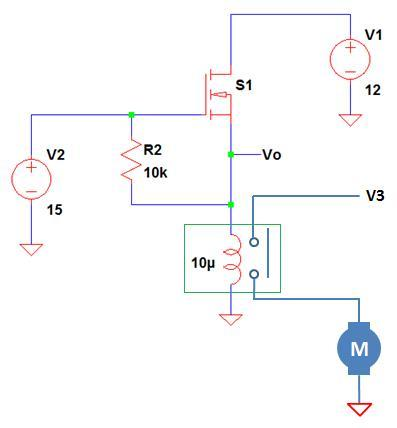Figure 2

Figure 2 shows a typical circuit for NMOS high side driver circuit. Again, the objective of the driving circuit is to energize the relay coil to close its contact and power up the motor load. The MOSFET S1 should be driven into saturation so that the voltage across the relay coil is more than enough to meet the required pickup voltage. The number one drawback of this configuration is that the gate needs a voltage higher than the level of V1. As a result, MCU output is not anymore applicable to this circuit. Let us analyze further how come.

As discussed above, for a NMOS to turn on, the gate to source voltage must be higher than the specified threshold voltage plus a margin. In this case, when the MOSFET saturates the level of source is also equal to V1. Now, when the value of V2 is lower than 10V; let us say 5V as previous section, the MOSFET will not turn on since VGS=5V-10V=-5V. Remember that for a NMOS the VGS must be positive. Now, you need to have another supply that is higher than V1 in order to drive the MOSFET into saturation and successfully turn on the relay. If your application is automotive, the nominal voltage for a car battery is around 13.5V and where do you get a supply that is higher than this. In typical application, we want to drive the gate of the MOSFET using MCU or DSP and with this configuration it is not possible.

1.3. High Side Driving Using PMOS

Another method in designing MOSFET switch particularly for high side driving is using PMOS. The two configurations we discussed so far are both using NMOS. In this section we will introduce PMOS. For NMOS, the gate source voltage is positive but for PMOS it is negative. If you will see the datasheet, the VGS threshold is negative. The more negative the gate to source the more the device will operate in saturation. Figure 3 illustrates a possible circuit for a high side driver using a PMOS.

Again the MOSFET should be operated at saturation in order to easily meet the pickup voltage requirement of the relay. Now let us analyze how this circuit operates. For S1 to turn on, its gate must be lower than the source. So, the gate must be zero so that the VGS is negative 12 (VGS=0V-12V=-12V). Since we need to pull the gate low in order S1 to turn on, thus we can use MCU because it can give a logic zero. The big question now is how to turn off the MOSFET. Since this is PMOS, the gate must be set equal to the source voltage to turn it off (VGS=0V). However you are using MCU to control the gate and the maximum logic high level the MCU can provide is only 5V. The source voltage is 12V and the gate voltage is 5V, it will give a VGS of -7V. A VGS of -7V is more than enough to keep the MOSFET ON since VGS are ranging from 1.5-4.5V depending on the device.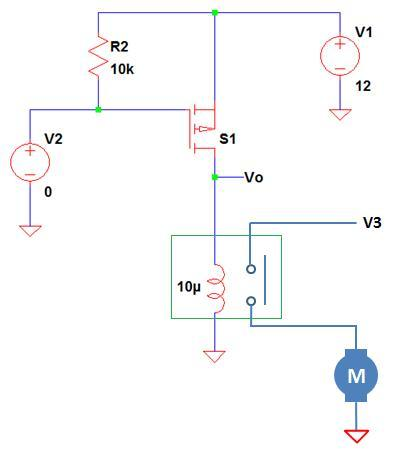Figure 3

If V2 is taken from an MCU output, S1 is therefore cannot be turned off. What if I will configure the MCU output as open collector or open drain? Yes you can turn off the MOSFET since VGS at this time is zero because the gate is pulled up by the resistor R2. However, the voltage seen in the gate is also 12V and this level will be experienced by the MCU pin and this is already a destructive level.

To improve the circuit, another low side driver may be added to drive the gate of the MOSFET from MCU output and this will make the circuit a bit complex and has added BOM cost.

To conclude this section, for a simple MOSFET relay driving circuit Figure 1 is the best choice. It is relatively simple and more flexible than the other two configurations. In some specific applications however, Figure 2 and Figure 3 circuit are can be used or improved version of it. However it will not be tackled in this paper anymore.

2. Designing MOSFET Switch – The Most Preferred Method for Relay Driving

Based from the comparison of the three circuit configurations, it is a smart move to use the first circuit. Figure 4 is just a copy of the first circuit.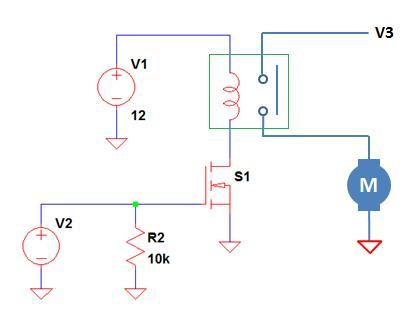Figure 4 – This is a low side relay driving circuit. When the relay contact closes, the front wiper motor starts to operate. V1 and V3 is 12V with +/-3% tolerance while V2 is derived from an MCU output which can give a maximum logic low level of 0.3V and a high level of 5V with +/-3%. The design will be exposed in an ambient temperature that is ranging from -40 to 120’C.

Now, we will start our objective which is designing MOSFET switch. We are going to use the circuit in Figure 4 for reference.

Step 1: Know the Circuit Requirements

As described above, the circuit is designed to drive a relay that is controlling an automobile front wiper motor. The voltages are given and also the operating temperature. From these specifications we can start the design.

Step 2: Select Initial Components

If you’re staring from the scratch, you can follow below steps for initial component selections. Why initial components? Because after we completed the circuit we are going to do detailed computations to cover all conditions like worst case and there we can see the stresses and know if we change another components.

2.1 Select a relay with a rated voltage equal or higher than the level of V1. In our assumption, V1 is 12V, so the relay is can be 12V rated as well. Also take note the operating temperature.

2.2 Select a higher coil resistance relay if you are planning to use a low power  MOSFET. A higher coil resistance will give smaller drain current and smaller  power dissipation on the part of the MOSFET.

2.3 Sizing the MOSFET by computing the drain current and the power dissipation using the typical values.

2.4 Verify the applied VGS if can make the MOSFET operate in saturation. The applied VGS should be higher than the gate to source threshold voltage of the MOSFET to drive it in saturation. For the value of R2, just put it 10kΩ or higher, it is not critical though.

3. Detailed Circuit Analysis

Now we will proceed to detailed and complete analysis. I will introduce to you “Worst Case Analysis”. This is the weapon used in high quality designs or products. With the term itself, it speaks of a worst case scenario; a worst case scenario your product may undergo. Is your product ready for this worst case scenario? I will teach you here so that your product will be very much ready even worse than worst case.

We start the analysis using below components:

Relay – G8JN Automotive Mini ISO relay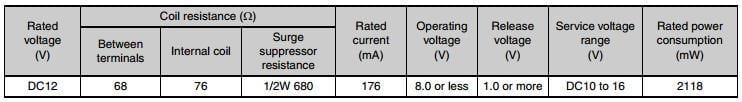Figure 5 – Coil DataFigure 6 – Relay other informations

MOSFET (S1) = MGSF1N03LT1 Power MOSFETFigure 7 – S1 maximum ratingsFigure 8 – S1 Thermal resistanceFigure 9 – Electrical Characteristics

We already selected a relay type and a particular MOSFET. As you observed, both are automotive grade since our application is automotive. For see the datasheets go to http://alldatasheet.com/.

3.1. Checking the Applied VGS if Enough to Saturate the MOSFET at Any Operating Temperature

The expectation of the MOSFET is to operate in the saturation so that the coil will be supplied with enough voltage to guarantee sure contact change state. Based from Figure 9, the gate to source threshold voltage is ranging from 1V, 1.7V and 2.4V for minimum, typical and maximum values respectively. However above values are taken when the drain current is 250µAdc. Based from Figure 5, the coil resistance of the relay between terminals is 68Ω and therefore this will give a typical drain current of 176mA (12V/68Ω). So, we will not use this data. We are going to use the information provided in VGS versus drain current graph as shown in Figure 10. The y-axis is the drain current while VGS is the x-axis. The horizontal red line is the 176mA drain current while the vertical dashed green, blue and red lines are the equivalent VGS at -55’C, 25’C and 150’C respectively.Figure 10

Based from Figure 10, at any operating temperature, the MOSFET will be driven into saturation because the minimum high voltage the MCU can give is 5V minus 3% which is 4.85V.

3.2. Compute the Coil Resistance of the Relay at Minimum, Typical and Maximum Operating Temperatures

Be careful with the data given in the relay specs since these are mostly taken at the typical ambient temperature or 20-25’C. In applications beyond the typical temperature, the coil resistance will change as well. There is no data about the coil resistance versus the ambient temperature on the datasheet of this relay. In case like this you can always send emails to the vendor and request as such. Another way is to use go back to physics and use the equation below.Equation 1

Where:

Rfinal is the resulting resistance due to the change in the operating temperature. Rinitial is the reference resistance while Tfinal is the temperature of interest other than the reference and Tinitial is the reference temperature.

Solving for the equivalent coil resistance at -40’C

As specified in Figure 4, the minimum operating temperature is -40’C. The equivalent coil resistance at this temperature level is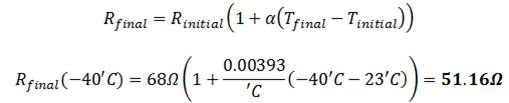Equation 2

(Most relay coil is made up from a copper material and copper has a temperature coefficient of resistance of 0.0039 per ‘C. Take note also that we use 25’C as the initial temperature. If the datasheet specified 20’C, use 20’C and so on.)

Solving for the equivalent coil resistance at 120’C

We will just repeat above procedure.Equation 3

3.3. Compute the Drain Current at Minimum, Typical and Maximum Operating Temperature

In step 3.1 we concluded that the MOSFET can operate in saturation at any temperature so the current that will flow in the drain of the MOSFET is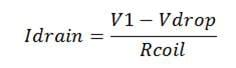Equation 4

Where:

Vdrop is the corresponding drop of the MOSFET due to its on state resistance. You can compute this value if you really want. However you can neglect this since this is very small in value and neglecting this one will simulate a worst case scenario which is okay to the design.

I mentioned earlier that we will consider here worst case, so for the above equation the worst is that the value of V1 is maximum and Vdrop is zero and the value of Rcoil is minimum. What we can get actually is the maximum drain current.

Solving for the drain current at -40’C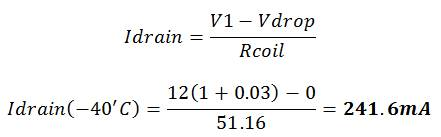Equation 5

(Note: V1 has a tolerance of +/-3% and since we are aiming for the maximum value, we consider the +3%. There is no tolerance specified for the coil resistance in the datasheet but if ever there is you must consider it also. Let say for example the specified tolerance is +/-10%, you must consider the negative tolerance and the coil resistance should be 51.16(1-0.1)=46.044).

Solving for the drain current at 25’CEquation 6

Solving for the drain current at 120’CEquation 7

3.4. Check the Current Stress of the MOSFET

Since we already get the maximum drain current of the MOSFET at the entire operating temperature range, we are now ready to check if there is no danger.

There is no information regarding the drain current rating versus the ambient temperature. Again, you can ask the vendor about this anytime. However, in Figure 7 the drain current is specified to be 2.1A at 25’C and 1.5A at 85’C. Most of the semiconductor devices have a de-rating behavior of a linear line with a negative slope wherein it starts to de-rate at 25’C (25’C is the typical or nominal ambient temperature specified by semiconductor vendor). So, based from this information, we can get the actual device current rating at 120’C. See below.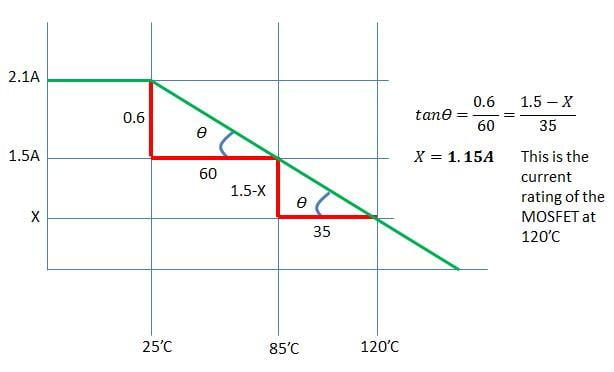Figure 11

from above derivation, the maximum current the MOSFET can allow at 120’C is 1.15A which is still very far from the actual current so there is no danger.

For 25’C and -40’C also, the current rating of the MOSFET is at its 100% which is 2.1A so the more the device cannot be damaged. By the way, from 25’C below, the current rating of the MOSFET is the same with the value at 25’C. It will only de-rate at temperature above 25’C.

3.5. Solving for On-state Resistance

Before we can solve for the power dissipation of the MOSFET, we need to get for the on state drain to source resistance first. This is a simple task because vendor always includes a graph of it on the datasheet. Figure 12 displays the equivalent on state resistance with respect to our operating temperatures. You have to take note that the graph is not showing an absolute value but it is a normalized one. This means that the initial Rdson (short for drain-source on state resistance) is to be multiplied by the value on the graph. So for -40’C, the multiplier is 0.8 while 1.4 for 120’C and the multiplier for 25’C is 1 since this is the reference for the normalization.

According to Figure 9, the maximum Rdson at nominal condition is 0.145ohm.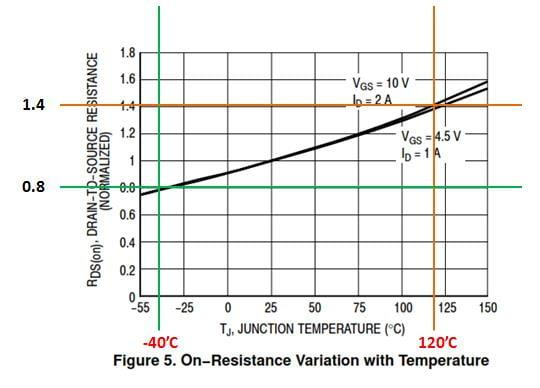Figure 12

3.6. Checking the Power Stress of the MOSFET

Some datasheet provides a graph of power dissipation versus ambient temperature and again you can ask the vendor for this if not given. For the transistor we used, there is no such graph. However we can solve for it anyway.

Solving for the actual power dissipation of the MOSFET at 25’C

The actual power dissipated by the MOSFET is just the product of the square of the drain current and the on-state resistance.Equation 8

Solving for the actual power dissipation of the MOSFET at -40’CEquation 9

Solving for the actual power dissipation of the MOSFET at 120’CEquation 10

According to Figure 7, the power dissipation (power rating of the device) at 25’C with the minimum recommended pad size is 0.42W. The computed power dissipation of the device at 25’C is only 4.7mW which is very far below this rating, thus at nominal ambient temperature operation there is no danger on the MOSFET.

At any temperature below the 25’C mark, the power dissipation rating of the device will be preserved. And hence, the computed value is very much below of the 0.42W rating, therefore at -40’C operation, the device will not be stressed.

What is left to be computed is the power rating of the device at the maximum ambient temperature of 120’C. The MOSFET rating will surely decrease and we will find out the new rating using below equation.Equation 11

Where:

Tjmax is the maximum junction temperature of the device specified in the datasheet. Tamax is the ambient temperature of interest while Rthja is the thermal resistance from junction to ambient.

The maximum operating junction temperature of the device we used is 150’C according to Figure 7. The thermal resistance junction to ambient with a minimum recommended pad size is 300’C/W. And now, the power dissipation capability of the MOSFET at 120’C isEquation 12

The actual power dissipated by the device at 120’C is only  which is very small compared to the rating which is 100mW. No doubt the device will not fail.

3.7. Checking if the Relay Can Surely Turn On at Any Operating Temperature

Every relay has a specified pickup or holdup voltage requirement. The datasheet of this relays uses the term operating voltage as shown in Figure 5 which is 8V or less. This means that there is a chance that the relay can turn on even the applied voltage is less than 8V. However, you have to take note that the worst case is that when the relay turn on with the highest voltage which is 8V. Therefore we will use 8V as the pickup voltage at the nominal ambient temperature which is 25’C in this discussion.

Since the operating ambient temperature is ranging from -40’C to 120’C, we need to consider as well how the pickup voltage varies with these temperatures. For the pickup voltage, you can request to the vendor for a graph of pickup voltage versus the operating ambient temperature. Alternatively, you can solve for it using the approach we use for the coil resistance.

Solving for the pickup voltage requirement at -40’C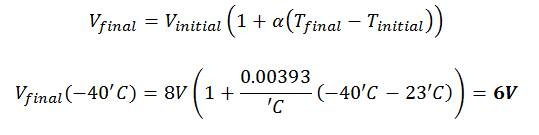Equation 13

Solving for the pickup voltage requirement at 120’CEquation 14

Now, we already solved for the relay coil voltage requirement for it to start operate normally, next is to find out if the applied voltage can provide this requirement. The applied voltage to the coil is the net voltage of V1 and the voltage drop of the MOSFET.

Solving for the voltage drop of the MOSFET at -40’C

The voltage drop of the MOSFET is just the product of the drain-source on state resistance (Rdson) and the current.Equation 15

Solving for the voltage drop of the MOSFET at 25’CEquation 16

Solving for the voltage drop of the MOSFET at 120’CEquation 17

Finally, we can now solve for the applied voltage to the relay coil.

Solving for the applied coil voltage at -40’CEquation 18

Take not that we used here the minimum level of V1 since this is the worst case.

Solving for the applied coil voltage at 25’CEquation 19

Solving for the applied coil voltage at 120’C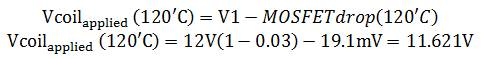Equation 20

Based from the computation results, the maximum voltage required for the coil to startup is 11.05V which is happening at the maximum temperature of 120’C. The computed minimum level the circuit can supply to the coil is 11.61V. Therefore there is no doubt that the relay can startup normally within the operating temperature range.

3.8. Checking the Maintaining Voltage across the Coil

The pickup or pull-in voltage requirement is for the relay to startup. When a relay is already running normally, the level of voltage it will need to maintain the contact at close condition (for a normally open relay) is may be less than the level of the pickup voltage. General purpose relay such as used in power supplies has 4V minimum voltage requirement while the pickup voltage nominal is 9V for a 12V rated device. This means that once the relay is already operating (after startup), the voltage it needs to maintain the contact state is just 4V.

In the relay we used here, the release voltage specified is 1V or more according to Figure 5. Since the voltage applied to the coil is fixed once the circuit is running normally, we can conclude that there is nothing to check with the maintaining voltage since we are providing a higher voltage all throughout.

3.9 Checking the Stress on the MOSFET at Turn Off

Relay coil is an inductor and it should follow the property of an inductor. Inductor will resist in a sudden change in current by producing a counter EMF. So, when the MOSFET is ON, the current will flow from V1 to the circuit ground and the relay coil will be energized. When this current is interrupted, the coil will reversed its polarity to maintain the same current and its direction in a short period of time. This action will generate a reversed voltage by which in nature is very high and if not consider will surely damage the MOSFET.

The analysis is somewhat difficult especially to those who are not really working in design for years. In practice, it is best to measure the actual kickback voltage experienced by the MOSFET at turn off then install a protection to avoid the circuit device from damage. However this approach is sometimes destructive especially when the level of the spike voltage is very high. I will demonstrate to you how to analyze and compute for the possible level of the MOSFET drain voltage at turn off so that we you have a point to start.Figure 13 – simplified version of our demo circuit wherein we did not include anymore the contact side of the relay since it is not relevant in this particular analysis.

At normal operation (say the MOSFET is operating at saturation and the relay coil is energized), the current will flow from V1 to the relay coil, to the MOSFET and to the circuit ground to complete the path. During this condition, the upper side of the relay coil (the side connected to V1) has a positive sign while the lower part of the coil has a negative sign as shown in the figure.

When the supply V2 is interrupted and the MOSFET will turn off, the relay coil will reversed its polarity to resist the change in current and try to maintain it in a short period of time. The circuit will now looks like below.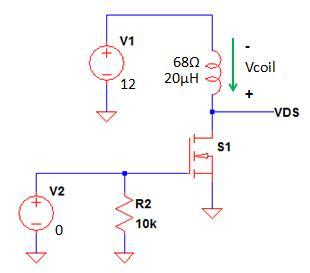Figure 14

Then the voltage seen in the VDS node is can be expressed by doing KVL from V1.Equation 21

Expressing VDS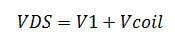Equation 22

Based from above equation, the voltage that MOSFET will see is the sum of the supply V1 and the coil voltage at the instance the current is interrupted. The voltage across an inductor is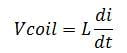Equation 23

So the VDS equation is can be re-written as below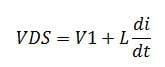Equation 24

Since we are accounting the instance the MOSFET is turned off, the current di during this time still the normal current that flow through the coil. However, the challenging part here is how to determine the time dt. I prepare an illustration in Figure 15 to discuss the determination of the time dt very well.Figure 15 – Voltage and current waveform of the MOSFET during turn off

The upper waveform of Figure 15 is the current on the MOSFET while the lower one is the VDS. While the MOSFET is running at saturation the current is steady and this is labeled as the running current in the figure. When the MOSFET is turned off, the current will start to drop and then oscillate until it will rest to zero. On the other hand, while the MOSFET is ON, the VDS is zero since the MOSFET is operating in saturation. When the MOSFET is off and the current starts to decay, the VDS will start to increase and has its maximum level when the current is zero.

Hence, it is clear that the change in current di is the difference of zero and the running current (0 – running current). And now the change in time (dt) that the current is changing from the running current value to zero is the difference between point A and B in the above figure. When we extend the point the running current starts to decay in such a way the same with the dashed orange line in the above figure (point C), we can have now sine wave signal with a period from point C to point E.

Where these oscillations come from? These oscillations are due to the resonance action of the coil inductance and output capacitance (Coss) of the MOSFET. A combination of L and C will always creates ringing. And now, if we can get the frequency from point C to point E, we can get the period and then we can get dt since it is just quarter of the period. But the question is how to get the frequency? The resonance frequency of L and C is can  be expressed using below equation.Equation 25

Where:

L is the coil inductance and C is the total drain capacitance which includes the layout and output capacitance of the MOSFET. If the layout is good, what will dominate is the Coss of the MOSFET. And also, a smaller value of C will give the worst case VDS, so the layout factor is may be neglected here.

Now, based from Figure 9 the output capacitance of the MOSFET is 100pF and we assumed a 20µH inductance for the relay coil, thus we can compute for the frequency as below.Equation 26

The period isEquation 27

And finally, dt isEquation 28

At last, the levels of VDS are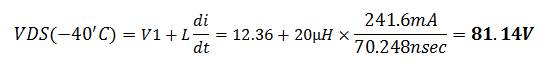Equation 29Equation 30

(Note: In Figure 15, the final current is zero and the initial current is the running current and the change in current di must be negative. However, we already accounted the negative sign into the coil voltage in our equation derivation, so the change current is no longer negative.)

From Figure 7, the maximum drain to source voltage (VDSS) the MOSFET can handle is only 30V hence it is expected to be damaged with the level of drain voltage which is reaching to 81.14V. To avoid damaging the device, you should install a protection. The most common protection is to use a freewheeling diode (Figure 16). Another method is to use a TVS across the MOSFET (Figure 17). The former has slower response then the latter. A diode with a 1A, 100V rating is already enough. For the TVS, the clamping voltage must be less than the minimum breakdown voltage of the MOSFET.Figure 16Figure 17

4. Conclusion

Above analysis in designing MOSFET switch is made for you to have an idea where to start. Some design engineers may have different approach in designing MOSFET switch. The bottom line is that as long as you can guarantee that at worst case condition your design can sustain, that’s it! Most of all, there is no substitute for actual testing. After you design through paper you should verify it in actual especially the VDS of the MOSFET during turn off because it is the trickiest part in the circuit design of relay driver. If you have something to add, feel free to share it under the comment section. Cheers!

1. Dattatray Jadhav says:

I am lucky that i got this link which improve and added in my knowledge..
so very very thank you..
but I have query regarding selection of TVS diode
1. How to select TVS diode for Spike like Load dump and lightning ..

2. Lindsay Newton says:

I am out of my depth but have been requested to find a circuit for a MOSFET switch with the following properties.
V = 1Kv A = 2-5A Frequency =5MHz Duty cycle =1-30% Must be neutral to polarity
Can you suggest a suitable circuit design ?

This site uses Akismet to reduce spam. Learn how your comment data is processed.